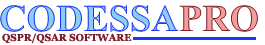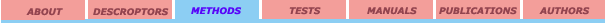Multilinear regression Selection of descriptors Multivariate methods

## Multilinear Regression

The basic method for QSPR analysis is essentially the solution of a multilinear regression problem. This can be expressed compactly and conveniently using matrix notation.[1, 2, 3] Suppose that there are n property values in Y and n associated calculated values for each k molecular descriptor in X columns. Then Yi, Xik, and ei can represent the ith value of the Y variable (property), the ith value of each of the X descriptors, and the ith unknown residual value, respectively. Collecting these terms into matrices we have:The multiple regression model in matrix notation then can be expressed as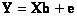where b is a column vector of coefficients (b1 is for the intercept) and k is the number unknown regression coefficients for the descriptors. We recall that the goal of multiple regression is to minimize the sum of the squared residuals: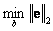Regression coefficients that satisfy this criterion are found by solving the system of linear equations (multiplying both sides by X’ from left)When the Xvariables are linearly independent (an X'X matrix which is of full rank), there is a unique solution to the system of linear equations. One of the ways for solving the system above is to premultiply both sides of the matrix formula for the normal equations by the inverse matrix X'X to give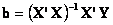The other way is to solve directly the system above using LS (underdetermined, n < k) or QR factorization for the overdetermined (n > k) system. This method is more general and does not require time-consuming matrix inversion. Singular value decomposition methods can also be used, but usually such methods are significantly more time-consuming and only advantageous when a strong linear dependence exists that would diminish quality of models.

The third way to solve the problem of linear dependency of variables (determinant of the X’X matrix is above zero) is by general matrix inversion, but this is usually outside the sphere of QSPR.

A fundamental principle of least squares methods, the multiple linear regression in particular, is that variance of the dependent variable can be partitioned (divided into parts) according to the source. Suppose that a dependent variable (property) is regressed on one or more descriptors and, for convenience, the dependent variable is scaled so that its mean is 0. Next, a basic least squares identity is calculated in which the total sum of squared values on the dependent variable equals the sum of squared predicted values plus the sum of squared residual values. Stated more generally,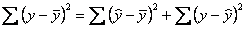where the term on the left is the total sum of squared deviations of the observed values on the dependent variable from the dependent variable mean, and the terms on the right are:

(i) the sum of the squared deviations of the predicted values for the dependent variable from the dependent variable mean and

(ii) the sum of the squared deviations of the observed values on the dependent variable from the predicted values, that is, the sum of the squared residuals.

Stated yet another way,Note that the SSTotal is always the same for any particular data set, but SSModel and the SSError vary with the regression equation. Assuming again that the dependent variable is scaled so that its mean is 0, the SSModeland SSError can be computed using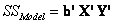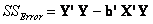Assuming that X'Xis full-rank,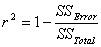where r2is squared correlation coefficient which is the measure of the quality of model fitnessto the property, s2 is an unbiased estimate of the residual or error variance, and F is Fisher criteria of (k, n - k - 1) degrees of freedom. If X'Xis not full rank, rank(X’X) + 1is substituted for k.

References:

1. Darlington, R. B. Regression and linear models. New York: McGraw-Hill, 1990.

2. Neter, J.; Wasserman, W.; Kutner, M. H. Applied linear regression models (2nd ed.). Homewood, IL: Irwin, 1989.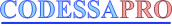University of Florida 2001. All rights reserved